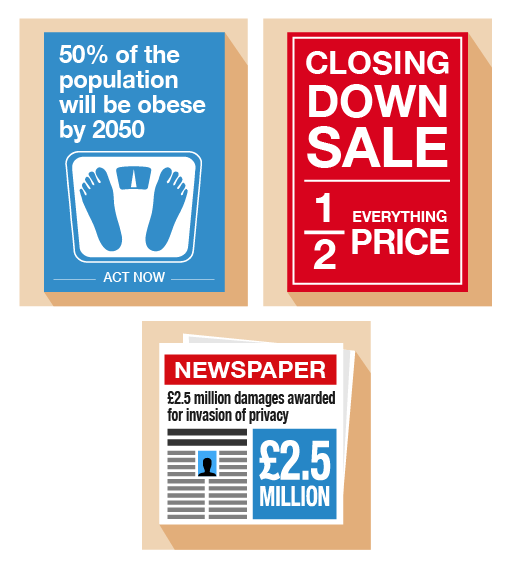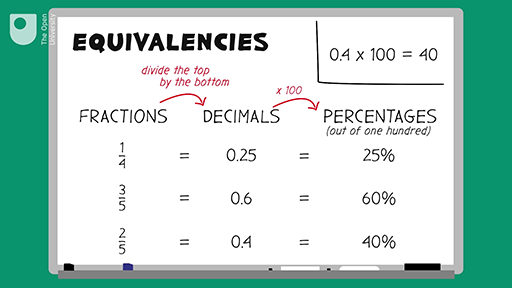Everyday maths 1

Start this free course now. Just create an account and sign in. Enrol and complete the course for a free statement of participation or digital badge if available.

Free course

# 6 Equivalencies between fractions, decimals and percentagesFigure 25 Looking at equivalencies

Fractions, decimals and percentages are different ways of saying the same thing. It’s an important skill to learn about the relationships (or ‘equivalencies’) between fractions, decimals and percentages to make sure you are getting the better deal.Skip transcript

#### Transcript

In this video, you’re going to look at equivalent fractions, decimals and percentages. First, let’s look at turning fractions into decimals. You have the fraction one quarter. To turn this into a decimal, you need to divide the top of the fraction by the bottom. 1 divided by 4 equals 0.25. Therefore, 0.25 is the equivalent decimal.

Let’s have a look at another fraction, three fifths. (Remember, divide the top of the fraction by the bottom.) 3 divided by 5 equals 0.6. So 0.6 is the equivalent decimal.

Now let’s try one last fraction see if you can calculate it before the answer is revealed! Two fifths: divide the top by the bottom. 2 divided by 5 equals 0.4. So the decimal is 0.4.

Now let’s use the decimals that we’ve calculated to find the percentage equivalent. A percentage is out of 100. Remember, ‘cent’ means 100. So to turn a decimal into a percentage, you need to multiply the decimal by 100.

For example, 0.25 times 100 equals 25. This is 25%.

0.6 times 100 equals 60, 60%.

0.4 times 100 equals 40. This is 40%.

Now, looking at the table, you can see how fractions, decimals and percentages relate to each other. You just need to remember the basic rules of turning a fraction into a decimal: you divide the top by the bottom, then, once you have the decimal, you can convert to per cent by multiplying by 100, remembering that ‘cent’ means 100.

Thank you for watching. Now you have a go.

End transcript

Interactive feature not available in single page view (see it in standard view).

Here are some common equivalencies. Try to memorise them – you will come across them a lot in everyday situations:

10% = = 0.1

20% = = 0.2

25% = = 0.25

50% = = 0.5

75% = = 0.75

100% = 1 = 1.0

Look at the following example. If you can identify equivalences, they’ll make it easier to make simple calculations.

## Example: Mine’s a half

What is 50% of £200?

### Method

Since 50% is the same as , so:

50% of £200 = of £200 = £100

## Activity 17: Looking for equivalencies

1. What is 20% of £600?
2. If you walked 0.25 km each day, what fraction of a kilometer have you walked?
3. House prices have increased by in the last five years. What is this increase as a percentage?
4. A DIY shop is holding a ‘50% off’ sale on kitchens. How much would you pay for a new kitchen worth £8,000 in the sale?
5. You buy an antique necklace for £3,000. After ten years, its value increases by 20%. How much is it now worth?

1. 20% is the same as .

• 600 ÷ 5 = 120

So 20% of £600 is £120.

2. 0.25 is the same as . There are 1,000 m in 1 km.

• 1,000 ÷ 4 = 250

So walking 0.25 km is the same as m, simplified to , or of a kilometre.

3. is the same as 50%.

So house prices have increased by 50% in the last five years.

4. 50% is the same as .

• 8,000 ÷ 2 = 4,000

The discount is £4,000, so the cost of a new kitchen worth £8,000 in the sale is:

• £8,000 – £4,000 = £4,000
5. 20% is the same as .

• 3,000 ÷ 5 = 600

The new value of necklace is:

• £3,000 + £600 = £3,600

Knowing the common equivalencies between fractions, decimals and percentages is important when trying to compare discounts when shopping or choosing a tariff when paying your bills.

## Summary

In this section you have learned about common equivalencies between fractions, decimals and percentages.

FSM_1